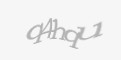# How to merge 2 tables into one result in MySQLToday I get interesting question. How to merge in MySQL two tables, where could be same primary key and different values. Result should be as select from one table with key column and 2 columns with both values from two tables, each in separate column.

Simply you can see what was requested on picture bellow.Lets start with solution, if you want you can follow my step by step with simple copy & paste each code step to you phpAdmin or other MySQL interface.

First step we need tables

```CREATE TABLE table1(
keyid varchar(50) primary key,
value int
);
CREATE TABLE table2(
keyid varchar(50) primary key,
value int
);
```

Second step, we need testing values same as in request to help

```INSERT INTO table1 (keyid, value)
VALUES ('a',2);
INSERT INTO table1 (keyid, value)
VALUES ('b',4);
INSERT INTO table1 (keyid, value)
VALUES ('c',3);
INSERT INTO table2 (keyid, value)
VALUES ('b',1);
INSERT INTO table2 (keyid, value)
VALUES ('c',3);
INSERT INTO table2 (keyid, value)
VALUES ('d',8);
```

Third step, check if data are in the tables. Just to be sure.

```SELECT keyid, value FROM table1;
``````SELECT keyid, value FROM table2;
```Now we can start with solution, first we need merge key values from both table, we will use UNION to do it.

```SELECT table1.keyid FROM table1
UNION
SELECT table2.keyid FROM table2
```You see, now he have list of keys.

Because we haven't for each key value in each table we cannot use simple JOIN but LEFT join to get data if they exist. One LEFT JOIN for table1 and second LEFT JOIN for table 2.

```SELECT tabkey.keyid, table1.value, table2.value
FROM
(SELECT table1.keyid FROM table1
UNION
SELECT table2.keyid FROM table2) as tabkey
LEFT JOIN
table1 on tabkey.keyid = table1.keyid
LEFT JOIN
table2 on tabkey.keyid = table2.keyid;
```We are almost done, there is kombinated keys in result, existing values are there, but NULL values in rows where value doesn't exists needs to be replaced by 0 as it was in request. This need last small modification by using IfNull function

#### Final query for MySQL

```SELECT tabkey.keyid, IfNull(table1.value, 0) as table1, IfNull(table2.value, 0) as table2
FROM
(SELECT table1.keyid FROM table1
UNION
SELECT table2.keyid FROM table2) as tabkey
LEFT JOIN
table1 on tabkey.keyid = table1.keyid
LEFT JOIN
table2 on tabkey.keyid = table2.keyid;
```

And here we are, result from final query:If you need same thing for MSSQL don't worry, T-SQL is slightly different but all you need change in final query is change IfNull to IsNull function and remove "as" before tabkey.

#### Final query with MSSQL modification

```SELECT tabkey.keyid, IsNull(table1.value, 0) as table1, IsNull(table2.value, 0)
as table2 FROM
(SELECT table1.keyid FROM table1
UNION
SELECT table2.keyid FROM table2) tabkey
LEFT JOIN
table1 on tabkey.keyid = table1.key
LEFT JOIN
table2 on tabkey.keyid = table2.key
```
Author info
Author: Stanislav DubenWebsite: http://www.duben.org